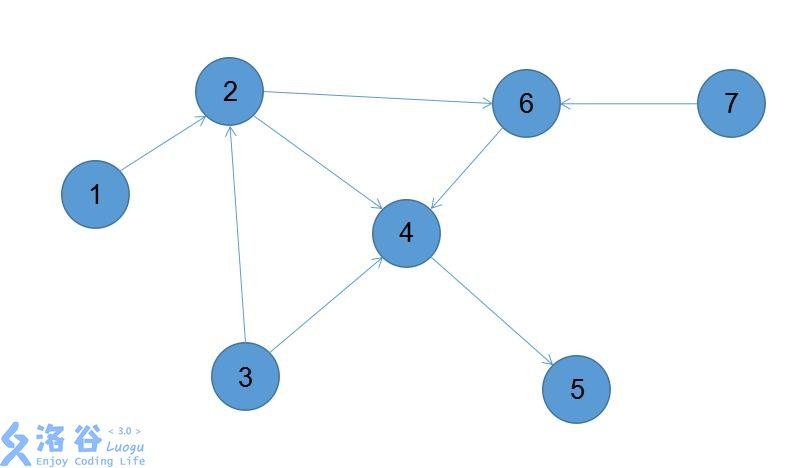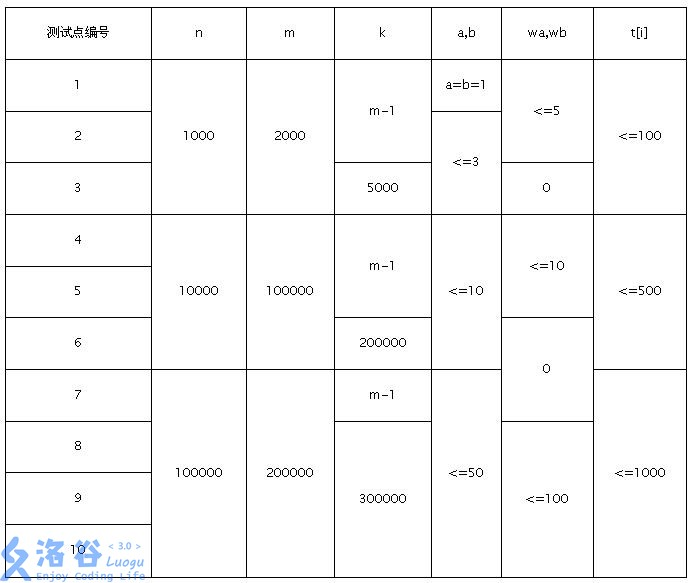P4918 信仰收集

• 68通过
• 380提交
• 题目提供者 沉迷学习的YSJ
• 评测方式 云端评测
• 标签
• 难度 省选/NOI-
• 时空限制 1000ms / 128MB
• 提示：收藏到任务计划后，可在首页查看。

题目背景

随着各种势力的迁入,守矢神社丧失了不少信仰

现在,为了挽回香火日益惨淡的神社,八坂神奈子派遣神社的风祝早苗去人类村落收集信仰

题目描述

你可以将村落看成一个$m$个点的有向无环图(编号从$1-m$),其中在某些点上有$n$簇待收集的信仰(每一簇都有一定的数量),图中有$k$有向边,每条边的长度均为$1$

早苗会从图中的$1$号点出发,在图中的任意一个点停止收集,当早苗在一个有信仰的点的时候,她会将这个点所有的信仰全部收集(包括$1$号点)

为了方便,早苗从宇佐见堇子那里学会了瞬移,所以她可以一次移动$a$个单位长度(称为小瞬移),也可以一次移动$b$个单位长度(称为大瞬移),分别会花费$wa$,$wb$点灵力,保证$a≤b$,但由于幻想乡不能被常识所束缚,所以$wa$不一定小于$wb$

现在,早苗希望你能帮她求出她在村落中能获得的(信仰数量-灵力耗费)的最大值

输入输出格式

输入格式：

第一行三个整数$n,m,k$,表示有信仰的点的数量,点的总数,有向边的条数
第二行两个整数$a,b$,表示两种瞬移的距离
第三行两个整数$wa,wb$,表示两种瞬移的灵力消耗

之后$n$行,每行两个正整数$pos,t$,表示每簇信仰所在的位置以及这簇信仰的数量,不保证$pos$互不相同

之后$k$行,每行两个整数$u,v$,表示从$u$到$v$有一条有向边

输出格式：

共一行一个整数$x$,表示最大的(信仰数量-灵力耗费)的最大值

输入输出样例

输入样例#1： 复制
3 7 8
1 2
3 2
2 2
4 3
6 4
1 2
2 4
4 5
2 6
7 6
6 4
3 2
3 4
输出样例#1： 复制
2

说明

样例$1$解释:
图如下所示:其中$2$号点有$2$信仰,$4$号点有$3$信仰,$6$号点有$4$信仰
早苗可以瞬移$1$或$2$条边,花费为$3,2$
最优的方案之一是从$1$瞬移到$6$花费$2$,收集了$6$号点的$4$点信仰后停止收集,信仰-消耗$=2$

数据范围:提示
标程仅供做题后或实在无思路时参考。
请自觉、自律地使用该功能并请对自己的学习负责。
如果发现恶意抄袭标程，将按照I类违反进行处理。